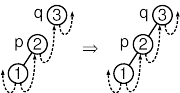7.11.1 From Tree to Vine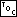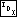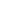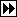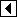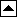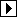We could transform a threaded binary tree into a vine in the same way we did for unthreaded binary trees, by use of rotations (see Transforming a BST into a Vine). But one of the reasons we did it that way was to avoid use of a stack, which is no longer a problem. It's now simpler to rearrange nodes by inorder traversal.

We start by finding the minimum node in the tree as p, which will step through the tree in inorder. During each trip through the main loop, we find p's successor as q and make p the left child of q. We also have to make sure that p's right thread points to q. That's all there is to it.

```284. <TBST tree-to-vine function 284> =
static void tree_to_vine (struct tbst_table *tree) {
struct tbst_node *p;

if (tree->tbst_root == NULL)
return;

p = tree->tbst_root;
while (p->tbst_tag == TBST_CHILD)

for (;;)     {
struct tbst_node *q = p->tbst_link;
if (p->tbst_tag == TBST_CHILD)         {
while (q->tbst_tag == TBST_CHILD)
}

if (q == NULL)
break;

q->tbst_tag = TBST_CHILD;
p = q;
}

tree->tbst_root = p;
}
```

This code is included in 282.

Sometimes one trip through the main loop above will put the TBST into an inconsistent state, where two different nodes are the parent of a third node. Such an inconsistency is always corrected in the next trip through the loop. An example is warranted. Suppose the original threaded binary tree looks like this, with nodes p and q for the initial iteration of the loop as marked: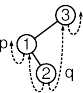The first trip through the loop makes p, 1, the child of q, 2, but p's former parent's left child pointer still points to p. We now have a situation where node 1 has two parents: both 2 and 3. This diagram tries to show the situation by omitting the line that would otherwise lead down from 3 to 2: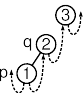On the other hand, node 2's right thread still points to 3, so on the next trip through the loop there is no trouble finding the new p's successor. Node 3 is made the parent of 2 and all is well. This diagram shows the new p and q, then the fixed-up vine. The only difference is that node 3 now, correctly, has 2 as its left child: